# Mean State

Period Mean (original grids) [K]
Model Period Mean (intersection) [K]
Model Period Mean (complement) [K]
Benchmark Period Mean (intersection) [K]
Benchmark Period Mean (complement) [K]
Bias [K]
RMSE [K]
Phase Shift [months]
Bias Score 
RMSE Score 
Seasonal Cycle Score 
Spatial Distribution Score 
Interannual Variability Score 
Overall Score 
Benchmark [-] 10.8
CLM4 [-] 12.5 12.6 7.83 10.9 8.01 1.49 1.99 0.750 0.313 0.457 0.891 0.985 0.549 0.609
CLM4.5 [-] 12.4 12.4 7.90 10.9 8.01 1.34 1.81 0.717 0.364 0.490 0.898 0.995 0.522 0.627
CLM5 [-] 12.0 12.0 7.44 10.9 8.01 0.911 1.41 0.537 0.424 0.556 0.925 0.974 0.497 0.656
Period Mean (original grids) [K]
Model Period Mean (intersection) [K]
Model Period Mean (complement) [K]
Benchmark Period Mean (intersection) [K]
Benchmark Period Mean (complement) [K]
Bias [K]
RMSE [K]
Phase Shift [months]
Bias Score 
RMSE Score 
Seasonal Cycle Score 
Spatial Distribution Score 
Interannual Variability Score 
Overall Score 
Benchmark [-] 13.8
CLM4 [-] 15.3 15.3 9.56 13.8 11.0 1.48 1.99 0.683 0.596 0.652 0.928 0.994 0.821 0.774
CLM4.5 [-] 14.9 14.9 9.40 13.8 11.0 1.06 1.59 0.685 0.690 0.696 0.929 0.960 0.794 0.794
CLM5 [-] 14.6 14.7 9.22 13.8 11.0 0.822 1.26 0.504 0.734 0.749 0.948 0.986 0.765 0.822
Period Mean (original grids) [K]
Model Period Mean (intersection) [K]
Model Period Mean (complement) [K]
Benchmark Period Mean (intersection) [K]
Benchmark Period Mean (complement) [K]
Bias [K]
RMSE [K]
Phase Shift [months]
Bias Score 
RMSE Score 
Seasonal Cycle Score 
Spatial Distribution Score 
Interannual Variability Score 
Overall Score 
Benchmark [-] 12.8
CLM4 [-] 14.4 14.5 7.06 12.8 9.07 1.29 1.86 0.412 0.445 0.605 0.951 0.981 0.738 0.721
CLM4.5 [-] 14.0 14.1 7.17 12.8 9.07 0.915 1.48 0.451 0.535 0.646 0.946 0.983 0.702 0.743
CLM5 [-] 13.9 14.0 6.81 12.8 9.07 0.800 1.35 0.410 0.549 0.696 0.951 0.981 0.687 0.760
Period Mean (original grids) [K]
Model Period Mean (intersection) [K]
Model Period Mean (complement) [K]
Benchmark Period Mean (intersection) [K]
Benchmark Period Mean (complement) [K]
Bias [K]
RMSE [K]
Phase Shift [months]
Bias Score 
RMSE Score 
Seasonal Cycle Score 
Spatial Distribution Score 
Interannual Variability Score 
Overall Score 
Benchmark [-] 9.39
CLM4 [-] 10.3 10.4 6.74 9.41 7.83 0.657 1.61 1.88 0.629 0.510 0.682 0.988 0.876 0.699
CLM4.5 [-] 10.2 10.3 6.74 9.41 7.83 0.614 1.60 1.67 0.637 0.513 0.718 0.985 0.882 0.708
CLM5 [-] 9.81 9.91 6.10 9.41 7.83 0.203 1.28 1.96 0.752 0.558 0.663 0.977 0.841 0.725
Period Mean (original grids) [K]
Model Period Mean (intersection) [K]
Model Period Mean (complement) [K]
Benchmark Period Mean (intersection) [K]
Benchmark Period Mean (complement) [K]
Bias [K]
RMSE [K]
Phase Shift [months]
Bias Score 
RMSE Score 
Seasonal Cycle Score 
Spatial Distribution Score 
Interannual Variability Score 
Overall Score 
Benchmark [-] 9.53
CLM4 [-] 10.4 10.7 7.44 9.60 8.86 0.408 1.90 1.02 0.180 0.415 0.866 0.982 0.714 0.595
CLM4.5 [-] 10.7 11.0 7.55 9.60 8.86 0.660 1.99 1.04 0.164 0.414 0.858 0.978 0.705 0.589
CLM5 [-] 10.2 10.4 7.01 9.60 8.86 0.116 1.72 0.900 0.224 0.492 0.876 0.963 0.763 0.635
Period Mean (original grids) [K]
Model Period Mean (intersection) [K]
Model Period Mean (complement) [K]
Benchmark Period Mean (intersection) [K]
Benchmark Period Mean (complement) [K]
Bias [K]
RMSE [K]
Phase Shift [months]
Bias Score 
RMSE Score 
Seasonal Cycle Score 
Spatial Distribution Score 
Interannual Variability Score 
Overall Score 
Benchmark [-] 10.7
CLM4 [-] 12.6 12.7 6.55 10.7 8.60 1.96 2.31 1.06 0.403 0.583 0.836 0.998 0.897 0.717
CLM4.5 [-] 12.1 12.2 6.74 10.7 8.60 1.46 1.94 0.880 0.512 0.586 0.878 0.966 0.913 0.740
CLM5 [-] 11.2 11.2 6.00 10.7 8.60 0.480 1.14 1.16 0.756 0.641 0.821 0.989 0.944 0.799
Period Mean (original grids) [K]
Model Period Mean (intersection) [K]
Model Period Mean (complement) [K]
Benchmark Period Mean (intersection) [K]
Benchmark Period Mean (complement) [K]
Bias [K]
RMSE [K]
Phase Shift [months]
Bias Score 
RMSE Score 
Seasonal Cycle Score 
Spatial Distribution Score 
Interannual Variability Score 
Overall Score 
Benchmark [-] 11.7
CLM4 [-] 13.1 13.2 7.53 11.8 9.57 1.21 1.73 0.799 0.550 0.589 0.885 0.997 0.849 0.743
CLM4.5 [-] 12.8 12.9 7.40 11.8 9.57 0.901 1.54 0.817 0.613 0.587 0.883 0.987 0.858 0.752
CLM5 [-] 12.2 12.3 6.69 11.8 9.57 0.292 1.08 0.681 0.737 0.639 0.905 0.999 0.916 0.806
Period Mean (original grids) [K]
Model Period Mean (intersection) [K]
Model Period Mean (complement) [K]
Benchmark Period Mean (intersection) [K]
Benchmark Period Mean (complement) [K]
Bias [K]
RMSE [K]
Phase Shift [months]
Bias Score 
RMSE Score 
Seasonal Cycle Score 
Spatial Distribution Score 
Interannual Variability Score 
Overall Score 
Benchmark [-] 11.1
CLM4 [-] 13.1 13.3 7.28 11.2 8.50 1.59 2.22 0.610 0.482 0.633 0.922 0.956 0.787 0.735
CLM4.5 [-] 12.7 12.8 7.38 11.2 8.50 1.18 1.79 0.508 0.561 0.672 0.938 0.979 0.835 0.776
CLM5 [-] 12.1 12.2 6.83 11.2 8.50 0.602 1.27 0.497 0.661 0.733 0.940 0.973 0.835 0.813
Period Mean (original grids) [K]
Model Period Mean (intersection) [K]
Model Period Mean (complement) [K]
Benchmark Period Mean (intersection) [K]
Benchmark Period Mean (complement) [K]
Bias [K]
RMSE [K]
Phase Shift [months]
Bias Score 
RMSE Score 
Seasonal Cycle Score 
Spatial Distribution Score 
Interannual Variability Score 
Overall Score 
Benchmark [-] 8.50
CLM4 [-] 9.26 9.35 6.29 8.51 7.45 0.703 1.51 0.910 0.645 0.545 0.861 0.962 0.868 0.738
CLM4.5 [-] 9.14 9.23 6.32 8.51 7.45 0.584 1.53 0.913 0.679 0.519 0.857 0.957 0.871 0.734
CLM5 [-] 8.59 8.67 5.72 8.51 7.45 0.0319 1.11 0.752 0.816 0.592 0.882 0.969 0.807 0.776
Period Mean (original grids) [K]
Model Period Mean (intersection) [K]
Model Period Mean (complement) [K]
Benchmark Period Mean (intersection) [K]
Benchmark Period Mean (complement) [K]
Bias [K]
RMSE [K]
Phase Shift [months]
Bias Score 
RMSE Score 
Seasonal Cycle Score 
Spatial Distribution Score 
Interannual Variability Score 
Overall Score 
Benchmark [-] 13.7
CLM4 [-] 15.1 15.2 7.62 13.8 10.4 0.967 1.57 0.389 0.515 0.669 0.963 0.939 0.878 0.772
CLM4.5 [-] 15.0 15.1 7.87 13.8 10.4 0.883 1.46 0.362 0.545 0.682 0.965 0.951 0.894 0.786
CLM5 [-] 14.7 14.8 7.39 13.8 10.4 0.595 1.25 0.385 0.603 0.706 0.964 0.932 0.913 0.804
Period Mean (original grids) [K]
Model Period Mean (intersection) [K]
Model Period Mean (complement) [K]
Benchmark Period Mean (intersection) [K]
Benchmark Period Mean (complement) [K]
Bias [K]
RMSE [K]
Phase Shift [months]
Bias Score 
RMSE Score 
Seasonal Cycle Score 
Spatial Distribution Score 
Interannual Variability Score 
Overall Score 
Benchmark [-] 8.57
CLM4 [-] 10.2 10.3 7.24 8.60 7.69 1.28 1.88 0.726 0.486 0.638 0.908 0.895 0.785 0.725
CLM4.5 [-] 10.3 10.4 7.53 8.60 7.69 1.37 1.94 0.831 0.473 0.621 0.895 0.937 0.777 0.721
CLM5 [-] 9.40 9.50 6.61 8.60 7.69 0.520 1.31 0.649 0.639 0.666 0.915 0.943 0.872 0.784
Period Mean (original grids) [K]
Model Period Mean (intersection) [K]
Model Period Mean (complement) [K]
Benchmark Period Mean (intersection) [K]
Benchmark Period Mean (complement) [K]
Bias [K]
RMSE [K]
Phase Shift [months]
Bias Score 
RMSE Score 
Seasonal Cycle Score 
Spatial Distribution Score 
Interannual Variability Score 
Overall Score 
Benchmark [-] 11.6
CLM4 [-] 12.6 13.2 7.02 11.8 7.98 1.15 1.85 0.779 0.478 0.580 0.891 0.993 0.788 0.718
CLM4.5 [-] 12.5 13.0 8.63 11.8 7.98 0.942 1.65 0.731 0.532 0.593 0.900 0.996 0.790 0.734
CLM5 [-] 12.0 12.6 7.84 11.8 7.98 0.472 1.27 0.655 0.629 0.646 0.910 0.994 0.799 0.771
Period Mean (original grids) [K]
Model Period Mean (intersection) [K]
Model Period Mean (complement) [K]
Benchmark Period Mean (intersection) [K]
Benchmark Period Mean (complement) [K]
Bias [K]
RMSE [K]
Phase Shift [months]
Bias Score 
RMSE Score 
Seasonal Cycle Score 
Spatial Distribution Score 
Interannual Variability Score 
Overall Score 
Benchmark [-] 14.0
CLM4 [-] 15.8 15.9 9.03 14.0 11.1 1.60 2.06 0.723 0.347 0.550 0.902 0.963 0.771 0.680
CLM4.5 [-] 15.6 15.6 8.89 14.0 11.1 1.33 1.82 0.650 0.398 0.575 0.912 0.971 0.759 0.698
CLM5 [-] 15.3 15.4 8.58 14.0 11.1 1.09 1.58 0.572 0.442 0.617 0.925 0.970 0.733 0.717
Period Mean (original grids) [K]
Model Period Mean (intersection) [K]
Model Period Mean (complement) [K]
Benchmark Period Mean (intersection) [K]
Benchmark Period Mean (complement) [K]
Bias [K]
RMSE [K]
Phase Shift [months]
Bias Score 
RMSE Score 
Seasonal Cycle Score 
Spatial Distribution Score 
Interannual Variability Score 
Overall Score 
Benchmark [-] 8.40
CLM4 [-] 9.41 9.50 6.50 8.41 7.44 0.795 1.61 1.33 0.568 0.495 0.784 0.943 0.902 0.698
CLM4.5 [-] 9.21 9.30 6.53 8.41 7.44 0.613 1.43 0.828 0.635 0.513 0.872 0.953 0.912 0.733
CLM5 [-] 8.58 8.67 5.85 8.41 7.44 -0.0175 1.07 0.851 0.794 0.573 0.867 0.971 0.906 0.781
Period Mean (original grids) [K]
Model Period Mean (intersection) [K]
Model Period Mean (complement) [K]
Benchmark Period Mean (intersection) [K]
Benchmark Period Mean (complement) [K]
Bias [K]
RMSE [K]
Phase Shift [months]
Bias Score 
RMSE Score 
Seasonal Cycle Score 
Spatial Distribution Score 
Interannual Variability Score 
Overall Score 
Benchmark [-] 13.3
CLM4 [-] 14.3 14.6 9.13 13.4 10.3 0.233 1.67 0.497 0.541 0.598 0.945 0.964 0.804 0.742
CLM4.5 [-] 14.2 14.5 9.34 13.4 10.3 0.211 1.60 0.545 0.552 0.598 0.938 0.981 0.814 0.747
CLM5 [-] 14.0 14.3 8.96 13.4 10.3 -0.00928 1.53 0.501 0.575 0.620 0.942 0.974 0.803 0.755
Period Mean (original grids) [K]
Model Period Mean (intersection) [K]
Model Period Mean (complement) [K]
Benchmark Period Mean (intersection) [K]
Benchmark Period Mean (complement) [K]
Bias [K]
RMSE [K]
Phase Shift [months]
Bias Score 
RMSE Score 
Seasonal Cycle Score 
Spatial Distribution Score 
Interannual Variability Score 
Overall Score 
Benchmark [-] 12.2
CLM4 [-] 13.2 13.2 9.96 12.2 9.97 0.880 1.39 1.12 0.554 0.627 0.824 0.901 0.885 0.736
CLM4.5 [-] 12.8 12.8 10.0 12.2 9.97 0.498 1.23 1.21 0.637 0.614 0.807 0.900 0.899 0.745
CLM5 [-] 12.2 12.2 9.22 12.2 9.97 -0.0749 0.948 0.838 0.752 0.656 0.871 0.911 0.943 0.798
Period Mean (original grids) [K]
Model Period Mean (intersection) [K]
Model Period Mean (complement) [K]
Benchmark Period Mean (intersection) [K]
Benchmark Period Mean (complement) [K]
Bias [K]
RMSE [K]
Phase Shift [months]
Bias Score 
RMSE Score 
Seasonal Cycle Score 
Spatial Distribution Score 
Interannual Variability Score 
Overall Score 
Benchmark [-] 11.0
CLM4 [-] 12.8 12.8 7.76 11.1 7.54 1.55 2.05 0.970 0.480 0.618 0.857 0.938 0.896 0.735
CLM4.5 [-] 12.4 12.4 7.84 11.1 7.54 1.18 1.79 0.853 0.537 0.622 0.876 0.976 0.917 0.758
CLM5 [-] 11.4 11.4 7.11 11.1 7.54 0.174 1.05 0.737 0.762 0.676 0.895 0.997 0.945 0.825
Period Mean (original grids) [K]
Model Period Mean (intersection) [K]
Model Period Mean (complement) [K]
Benchmark Period Mean (intersection) [K]
Benchmark Period Mean (complement) [K]
Bias [K]
RMSE [K]
Phase Shift [months]
Bias Score 
RMSE Score 
Seasonal Cycle Score 
Spatial Distribution Score 
Interannual Variability Score 
Overall Score 
Benchmark [-] 15.3
CLM4 [-] 15.7 15.7 10.0 15.3 11.3 0.289 1.29 0.500 0.740 0.674 0.938 0.995 0.897 0.820
CLM4.5 [-] 15.4 15.4 10.6 15.3 11.3 -0.0196 1.30 0.509 0.749 0.654 0.936 0.992 0.899 0.814
CLM5 [-] 15.1 15.1 9.64 15.3 11.3 -0.320 1.20 0.455 0.769 0.663 0.946 0.999 0.931 0.828
Period Mean (original grids) [K]
Model Period Mean (intersection) [K]
Model Period Mean (complement) [K]
Benchmark Period Mean (intersection) [K]
Benchmark Period Mean (complement) [K]
Bias [K]
RMSE [K]
Phase Shift [months]
Bias Score 
RMSE Score 
Seasonal Cycle Score 
Spatial Distribution Score 
Interannual Variability Score 
Overall Score 
Benchmark [-] 10.2
CLM4 [-] 11.5 11.5 7.44 10.2 9.50 1.18 1.55 0.525 0.385 0.552 0.937 0.989 0.516 0.655
CLM4.5 [-] 11.6 11.7 7.70 10.2 9.50 1.31 1.62 0.532 0.368 0.572 0.940 0.992 0.510 0.659
CLM5 [-] 11.1 11.1 7.07 10.2 9.50 0.792 1.12 0.433 0.493 0.656 0.948 0.991 0.463 0.701
Period Mean (original grids) [K]
Model Period Mean (intersection) [K]
Model Period Mean (complement) [K]
Benchmark Period Mean (intersection) [K]
Benchmark Period Mean (complement) [K]
Bias [K]
RMSE [K]
Phase Shift [months]
Bias Score 
RMSE Score 
Seasonal Cycle Score 
Spatial Distribution Score 
Interannual Variability Score 
Overall Score 
Benchmark [-] 11.7
CLM4 [-] 12.9 13.0 7.46 11.7 9.16 1.19 1.58 0.497 0.450 0.573 0.939 0.980 0.605 0.687
CLM4.5 [-] 12.7 12.8 7.74 11.7 9.16 0.962 1.32 0.428 0.517 0.609 0.947 0.993 0.627 0.717
CLM5 [-] 12.4 12.5 7.16 11.7 9.16 0.663 1.02 0.367 0.600 0.687 0.955 0.985 0.632 0.758
Period Mean (original grids) [K]
Model Period Mean (intersection) [K]
Model Period Mean (complement) [K]
Benchmark Period Mean (intersection) [K]
Benchmark Period Mean (complement) [K]
Bias [K]
RMSE [K]
Phase Shift [months]
Bias Score 
RMSE Score 
Seasonal Cycle Score 
Spatial Distribution Score 
Interannual Variability Score 
Overall Score 
Benchmark [-] 12.5
CLM4 [-] 14.2 14.2 9.04 12.6 7.59 0.981 1.82 0.918 0.496 0.582 0.885 0.975 0.768 0.715
CLM4.5 [-] 13.8 13.8 8.94 12.6 7.59 0.611 1.59 0.929 0.564 0.583 0.877 0.985 0.761 0.725
CLM5 [-] 13.7 13.7 8.63 12.6 7.59 0.457 1.44 0.845 0.595 0.615 0.897 0.976 0.743 0.740

# Temporally integrated period mean

BENCHMARK MEAN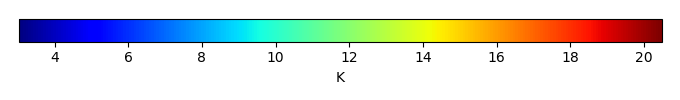MODEL MEANBIAS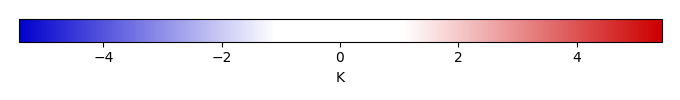BIAS SCORERMSE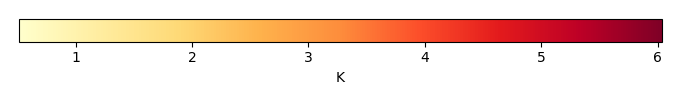RMSE SCOREBENCHMARK INTERANNUAL VARIABILITY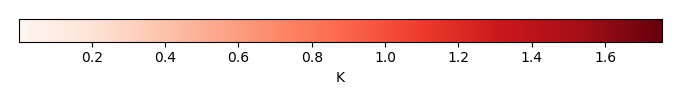MODEL INTERANNUAL VARIABILITYINTERANNUAL VARIABILITY SCOREBENCHMARK MAX MONTHMODEL MAX MONTHDIFFERENCE IN MAX MONTH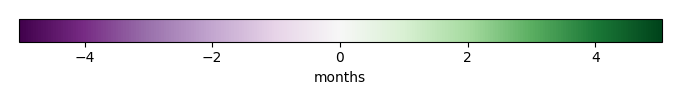SEASONAL CYCLE SCORESPATIAL TAYLOR DIAGRAMMODEL COLORS# Spatially integrated regional mean

MODEL COLORSREGIONAL MEANANNUAL CYCLEMONTHLY ANOMALYANNUAL CYCLE# All Models

BenchmarkCLM4CLM4.5CLM5# Data Information

creation_date: Fri Feb 8 13:55:45 PST 2019

history: 2018-01-15: download from https://crudata.uea.ac.uk/cru/data/hrg/cru_ts_4.01/cruts.1709081022.v4.01/ 2019-02-06: converted and created to netCDF4 for ILAMB package

original_resolution: 0.5x0.5

resolution: 0.5x0.5

original_frequency: mon

frequency: mon

references: Harris et al., 2014, Int. J. Climatol., doi:10.1002/joc.3711

institution: Original Data held at British Atmospheric Data Centre, RAL, UK.

source: Run ID = 1709081022. Data generated from:dtr.1708250000.dtb

title: CRU TS4.01 Diurnal Temperature Range

Conventions: CF-1.7